Views:
Question:
How can I use workflow to determine the lowest number in a keyword or table field?

Please refer to the following demonstration on how to determine the lowest value contained in a keyword field.
Since Table fields are comprised of keywords, this would also applicable to these fields as well.

The following image is the overall workflow build and should serve as a template on how this could be setup.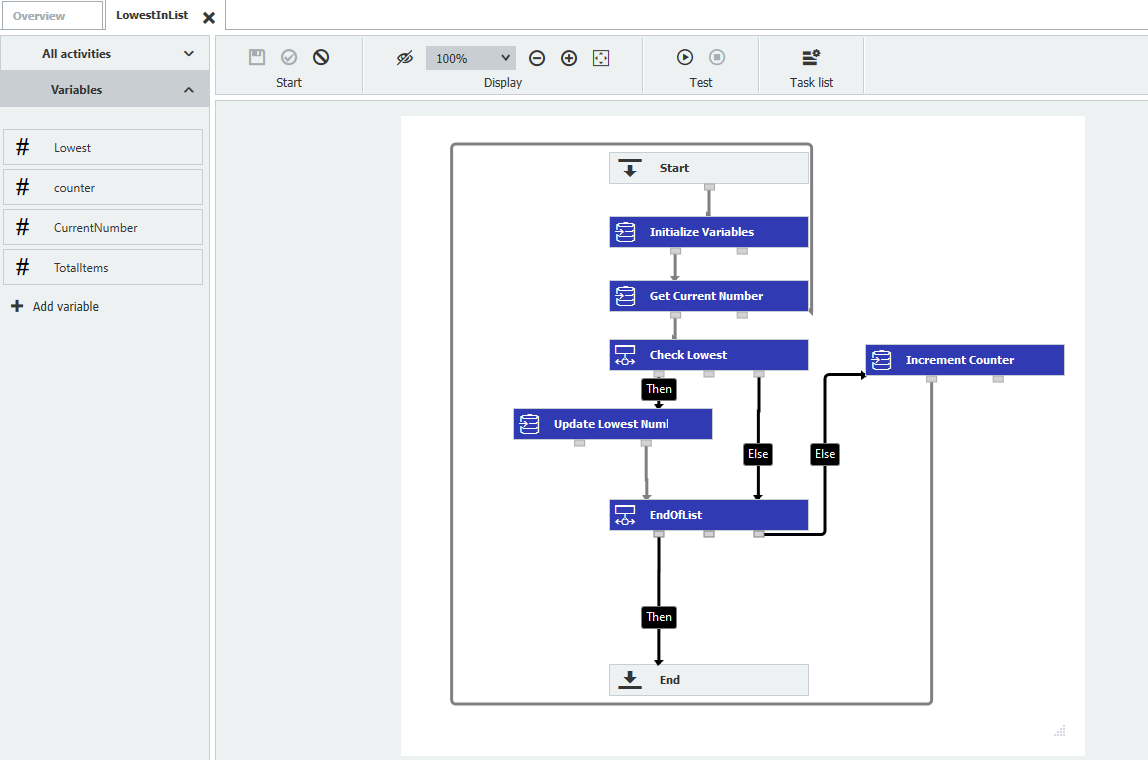1. Initialize Variables
The four following Global variables will need to be created and initialized during this step. Each variable will need to be created as an Integer data type.

Counter = Fixed Entry of 0
TotalItems = Arithmetic Expression of COUNT(Keyword Field)
CurrentNumber = Arithmetic Expression of cInt(Keyword Field[GV_counter])
Lowest = Global Variable CurrentNumber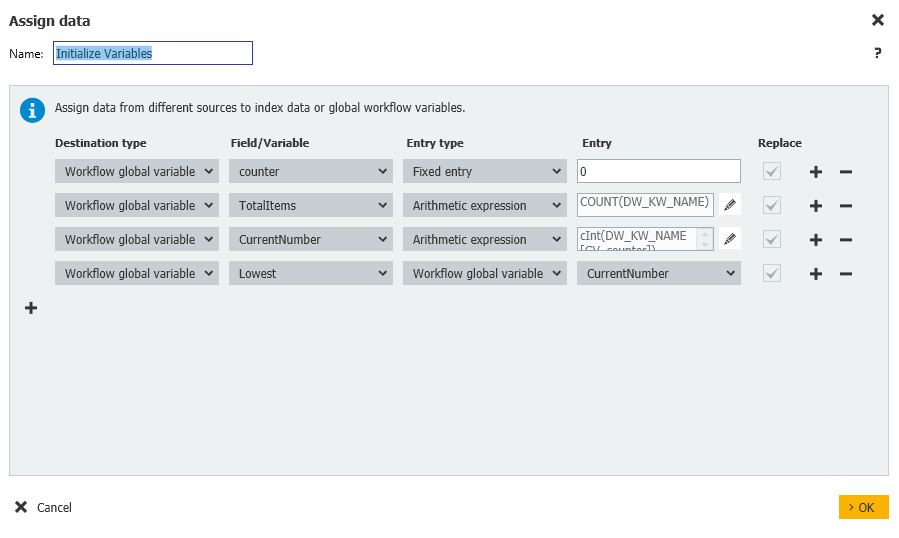2. Get Current Number
On this step, we need to re-set the current item of the list using the following assignment;

CurrentNumber = Arithmetic Expression of cInt(Keyword Field[GV_counter])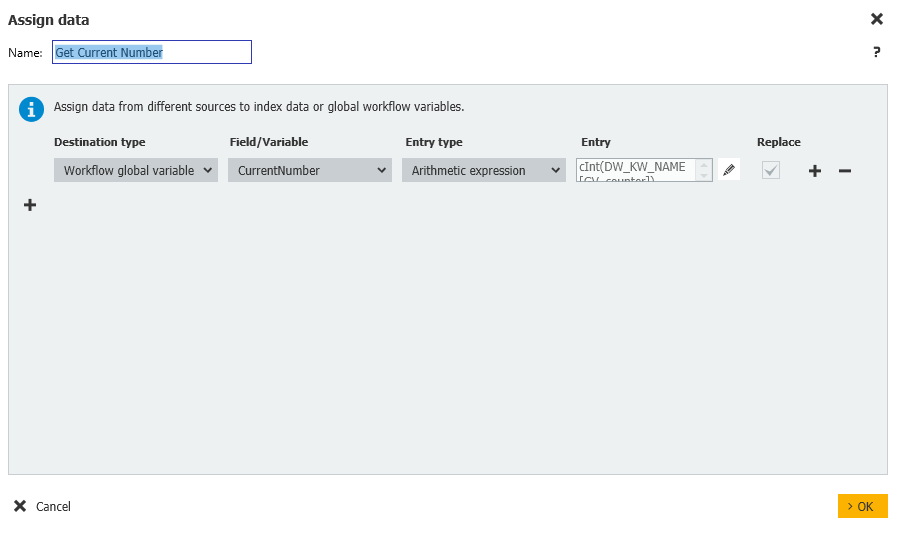3. Check for the lowest number
During the Condition step, we now check whether our current number is lower than our currently set Lowest variable.
If it is, we will update the Lowest variable, otherwise, we'll skip to checking if we're at the end of the list yet.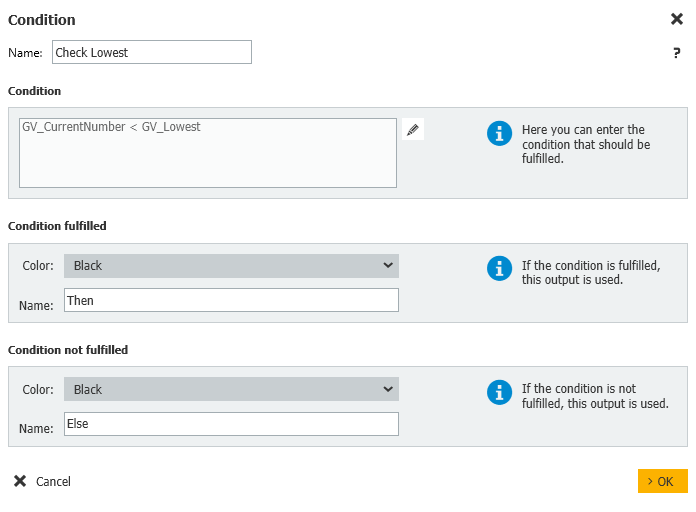4. Update the Lowest Number
If our current number is lower, then we'll update the lowest variable with the following,

Lowest = Global Variable of CurrentNumber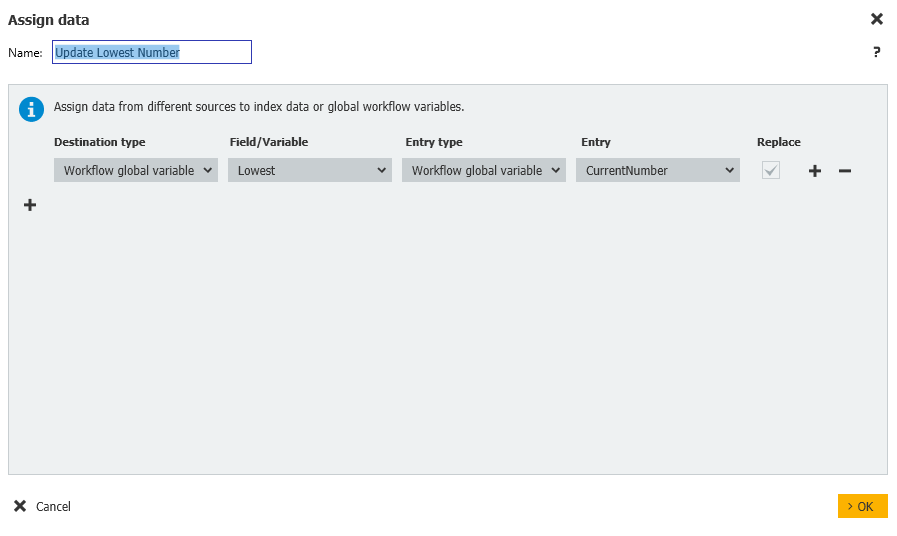5. Check if at the end of the list
Here, we'll check the TotalItem variable to determine whether we have more items to process.
If we do, we'll increment the counter, then perform the steps above again. Otherwise, we'll exit the workflow.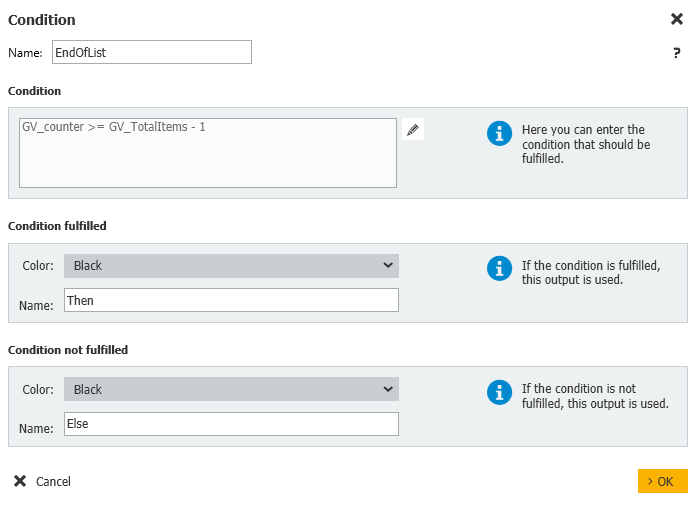6. Increment the Counter
If we have more to process, this Assign Data step will increment the counter by 1.

Counter = Arithmetic Expression of GV_Counter + 1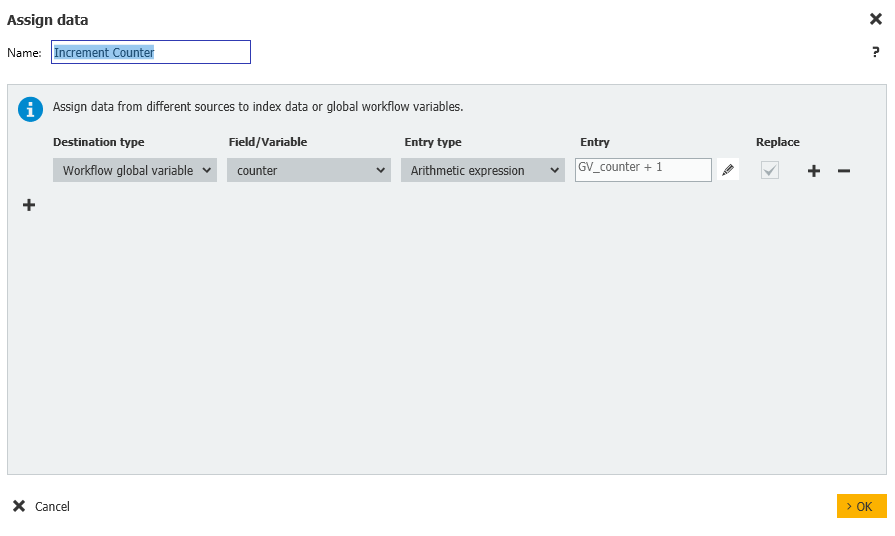7. If nothing more, exit the workflow.

KBA applicable for both Cloud and On-premise Organizations.